# Linear Algebra FREE

## The most powerful mathematical app!

4.29 EDUCATION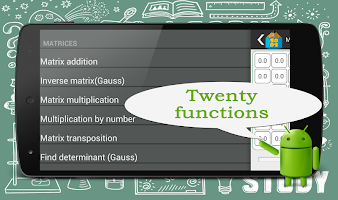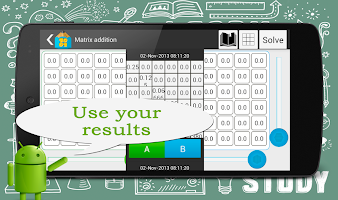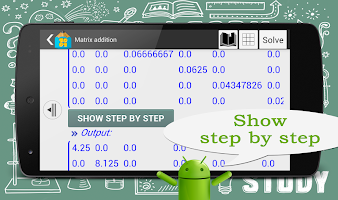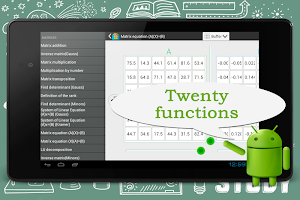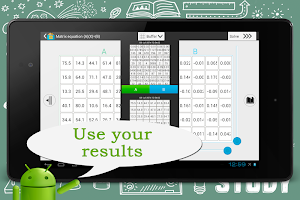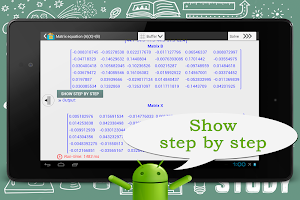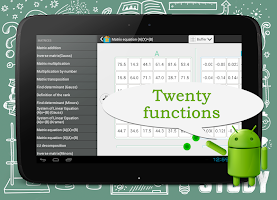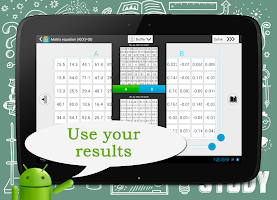The most powerful mathematical app!
Free “Step by step” for all functions.

ALL AVAILABLE FUNCTIONS:
---Matrix algebra---
[+] inverse
[+] matrix multiplication
[+] multiplication by number
[+] matrix transposition
[+] find determinant (Gauss)
[+] definition of the rank
[+] find determinant (Minors)
[+] System Of Linear Equation (A)x=(B) (Gauss)
[+] System Of Linear Equation (A)x=(B) (Kramer)
[+] matrix equation (A)(X)=(B)
[+] matrix equation (X)(A)=(B)
[+] LU decomposition
[+] inverse matrix (Minors)
[+] matrix power
[+] eigenvalues
---Vector algebra---
[+] dot product
[+] length of vector
[+] cross product
[+] angle between vectors
[+] build vector by points

FEATURES:
[-] theory
[-] new functions (vector algebra)
[-] export to Maple, MathLab, Wolfram
[-] clipboard for cells

Download assistant for solving problems in areas such as: linear algebra, analysis, economics, differential equations and computer graphics.

- New interface with new design - Vector algebra - Clipboard for matrices - Speed up Math kernel - More bugs fixed - Support most devices with Android 2.3 - 4.4

### Details and Recent Ratings

Size: 0 MB
Version: 2.0 by Artem Grinko
Updated: 05 November 2013 (3164 days ago)
Released: 11 November 2012
Installations: more than 50 000
5 Stars: 526
4 Stars: 209
3 Stars: 101
2 Stars: 0
1 Star: 47

### Similar apps

Android Game Categories
Android App Categories